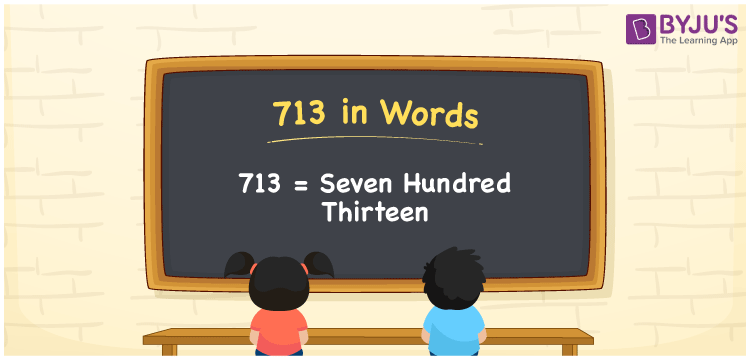# 713 in words

713 in words is written as Seven Hundred and Thirteen. 713 represents the count or value. The article on Counting Numbers can give you an idea about count or counting. The number 713 is a 3 digit number that is used in expressions related to money, days, distance, length, weight and so on. Let us consider an example for 713. “I am yet to read Seven Hundred and Thirteen more pages from a novel.”

 713 in words Seven Hundred and Thirteen Seven Hundred and Thirteen in Numbers 713

## 713 in English Words## How to Write 713 in Words?

We can convert 713 to words using a place value chart. The number 713 has 3 digits, so let’s make a chart that shows the place value up to 3 digits.

 Hundreds Tens Ones 7 1 3

Thus, we can write the expanded form as:

7 × Hundred + 1 × Ten + 3 × One

= 7 × 100 + 1 × 10 + 3 × 1

= 713

= Seven Hundred and Thirteen.

713 is the natural number that is succeeded by 712 and preceded by 714.

713 in words – Seven Hundred and Thirteen.

Is 713 an odd number? – Yes.

Is 713 an even number? – No.

Is 713 a perfect square number? – No.

Is 713 a perfect cube number? – No.

Is 713 a prime number? – No.

Is 713 a composite number? – Yes.

## Solved Example

1. Write the number 713 in expanded form

Solution: 7 × 100 + 1 × 10 + 3 × 1

We can write 713 = 700 + 10 + 3

= 7 × 100 + 1 × 10 + 3 × 1.

## Frequently Asked Questions on 713 in words

Q1

### How to write the number 713 in words?

713 in words is written as Seven Hundred and Thirteen.
Q2

### Is 713 divisible by 3?

No. 713 is not divisible by 3.
Q3

### Is 713 a perfect square number?

No. 713 is not a perfect square number.